# OpenCV-Python教程（4、形态学处理）

• 转载请详细注明原作者及出处，谢谢！
• 本文介绍使用OpenCV-Python进行形态学处理
• 本文不介绍形态学处理的基本概念，所以读者需要预先对其有一定的了解。

# 定义结构元素

element = cv2.getStructuringElement(cv2.MORPH_CROSS,(5,5))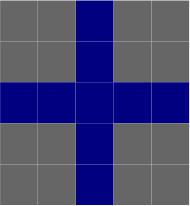NpKernel = np.uint8(np.zeros((5,5)))
for i in range(5):
NpKernel[2, i] = 1 #感谢chenpingjun1990的提醒，现在是正确的
NpKernel[i, 2] = 1

[[0 0 1 0 0]
[0 0 1 0 0]
[1 1 1 1 1]
[0 0 1 0 0]
[0 0 1 0 0]]## 腐蚀和膨胀

#coding=utf-8
import cv2
import numpy as np

#OpenCV定义的结构元素
kernel = cv2.getStructuringElement(cv2.MORPH_RECT,(3, 3))

#腐蚀图像
eroded = cv2.erode(img,kernel)
#显示腐蚀后的图像
cv2.imshow("Eroded Image",eroded);

#膨胀图像
dilated = cv2.dilate(img,kernel)
#显示膨胀后的图像
cv2.imshow("Dilated Image",dilated);
#原图像
cv2.imshow("Origin", img)

#NumPy定义的结构元素
NpKernel = np.uint8(np.ones((3,3)))
Nperoded = cv2.erode(img,NpKernel)
#显示腐蚀后的图像
cv2.imshow("Eroded by NumPy kernel",Nperoded);

cv2.waitKey(0)
cv2.destroyAllWindows()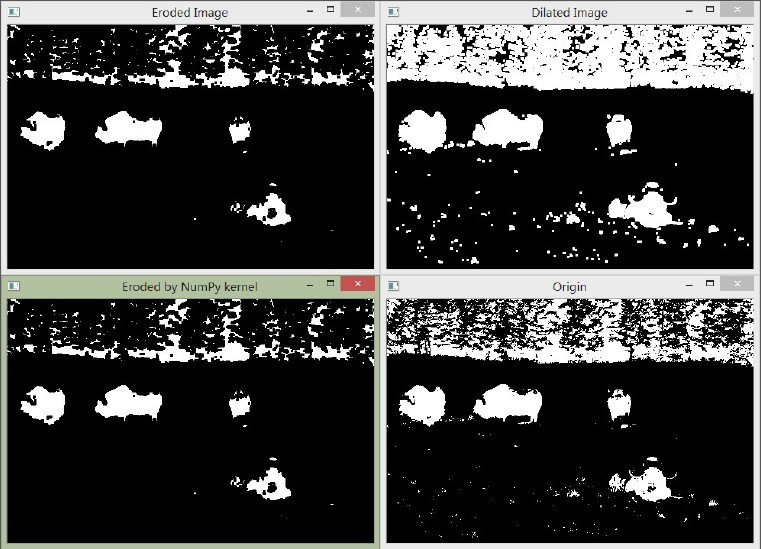## 开运算和闭运算

#coding=utf-8
import cv2
import numpy as np

#定义结构元素
kernel = cv2.getStructuringElement(cv2.MORPH_RECT,(5, 5))

#闭运算
closed = cv2.morphologyEx(img, cv2.MORPH_CLOSE, kernel)
#显示腐蚀后的图像
cv2.imshow("Close",closed);

#开运算
opened = cv2.morphologyEx(img, cv2.MORPH_OPEN, kernel)
#显示腐蚀后的图像
cv2.imshow("Open", opened);

cv2.waitKey(0)
cv2.destroyAllWindows()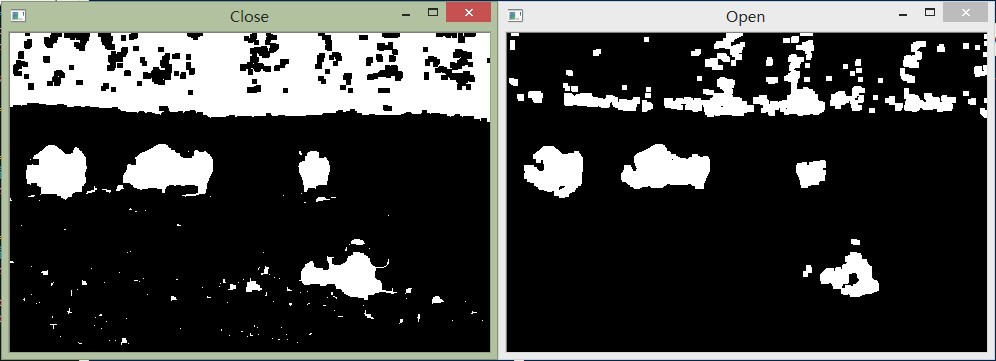## 用形态学运算检测边和角点

### 检测边缘

#coding=utf-8
import cv2
import numpy

#构造一个3×3的结构元素
element = cv2.getStructuringElement(cv2.MORPH_RECT,(3, 3))
dilate = cv2.dilate(image, element)
erode = cv2.erode(image, element)

#将两幅图像相减获得边，第一个参数是膨胀后的图像，第二个参数是腐蚀后的图像
result = cv2.absdiff(dilate,erode);

#上面得到的结果是灰度图，将其二值化以便更清楚的观察结果
retval, result = cv2.threshold(result, 40, 255, cv2.THRESH_BINARY);
#反色，即对二值图每个像素取反
result = cv2.bitwise_not(result);
#显示图像
cv2.imshow("result",result);
cv2.waitKey(0)
cv2.destroyAllWindows()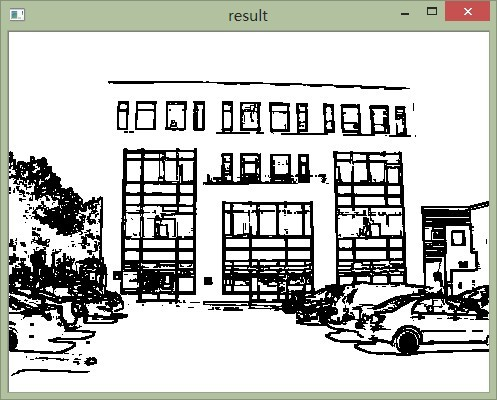### 检测拐角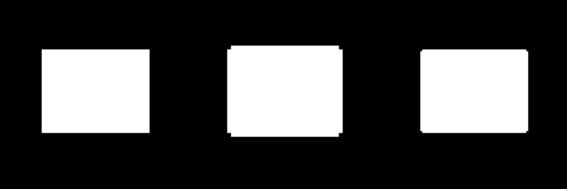#coding=utf-8
import cv2

image = cv2.imread("D:/building.jpg", 0)
#构造5×5的结构元素，分别为十字形、菱形、方形和X型
cross = cv2.getStructuringElement(cv2.MORPH_CROSS,(5, 5))
#菱形结构元素的定义稍麻烦一些
diamond = cv2.getStructuringElement(cv2.MORPH_RECT,(5, 5))
diamond[0, 0] = 0
diamond[0, 1] = 0
diamond[1, 0] = 0
diamond[4, 4] = 0
diamond[4, 3] = 0
diamond[3, 4] = 0
diamond[4, 0] = 0
diamond[4, 1] = 0
diamond[3, 0] = 0
diamond[0, 3] = 0
diamond[0, 4] = 0
diamond[1, 4] = 0
square = cv2.getStructuringElement(cv2.MORPH_RECT,(5, 5))
x = cv2.getStructuringElement(cv2.MORPH_CROSS,(5, 5))
#使用cross膨胀图像
result1 = cv2.dilate(image,cross)
#使用菱形腐蚀图像
result1 = cv2.erode(result1, diamond)

#使用X膨胀原图像
result2 = cv2.dilate(image, x)
#使用方形腐蚀图像
result2 = cv2.erode(result2,square)

#result = result1.copy()
#将两幅闭运算的图像相减获得角
result = cv2.absdiff(result2, result1)
#使用阈值获得二值图
retval, result = cv2.threshold(result, 40, 255, cv2.THRESH_BINARY)

#在原图上用半径为5的圆圈将点标出。
for j in range(result.size):
y = j / result.shape
x = j % result.shape

if result[x, y] == 255:
cv2.circle(image, (y, x), 5, (255,0,0))

cv2.imshow("Result", image)
cv2.waitKey(0)
cv2.destroyAllWindows()

cv2.circle(image, (5, 10), 5, (255,0,0))
image[5, 10] = 0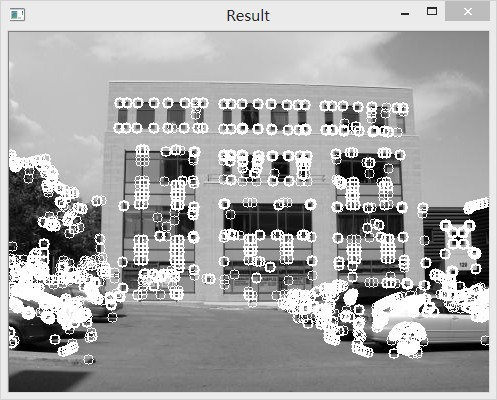## 参考资料：

1、《Opencv2 Computer Vision Application Programming Cookbook》

2、《OpenCV References Manule》